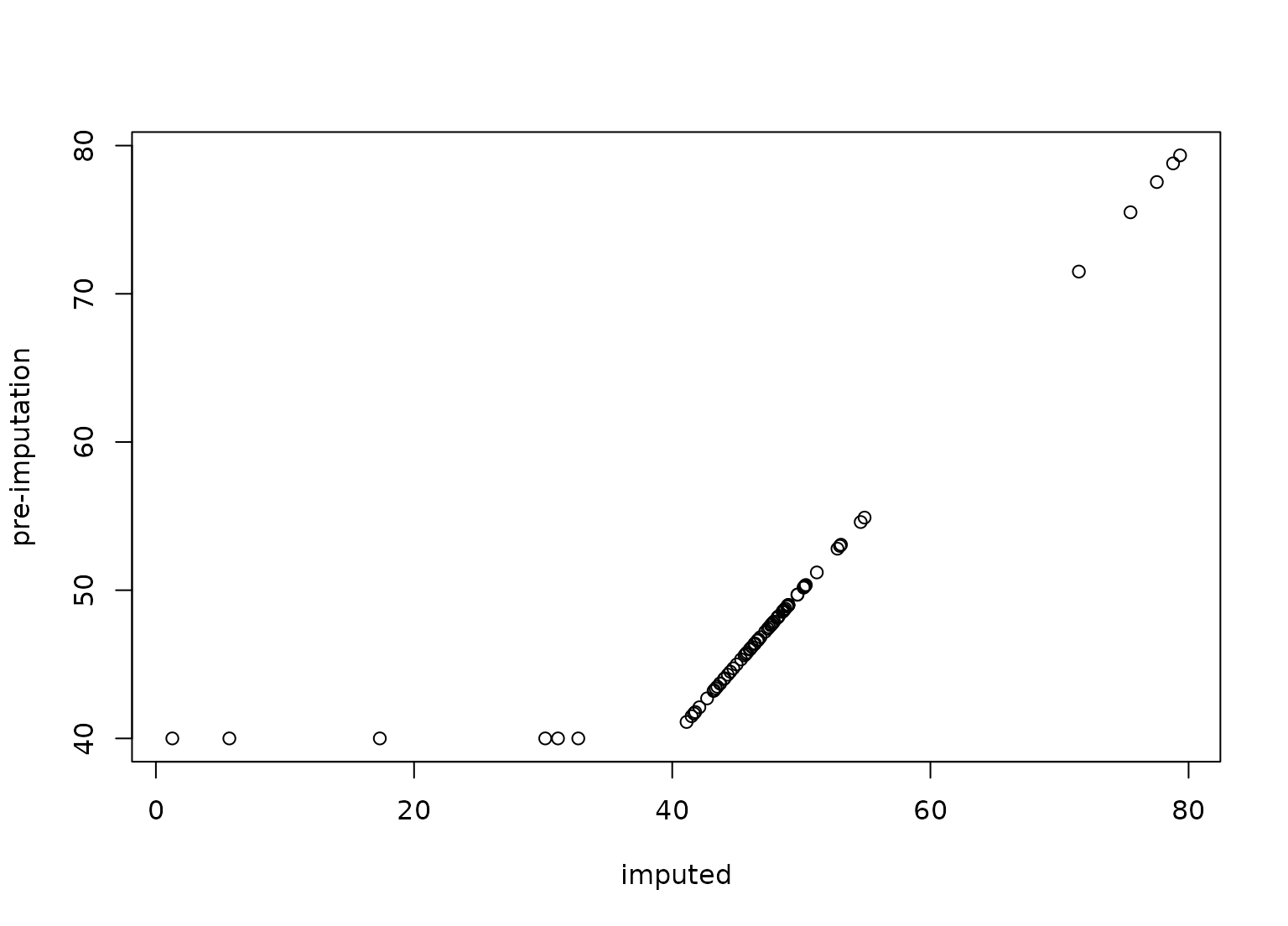step_impute_lower() creates a specification of a recipe step designed for cases where the non-negative numeric data cannot be measured below a known value. In these cases, one method for imputing the data is to substitute the truncated value by a random uniform number between zero and the truncation point.

## Usage

step_impute_lower(
recipe,
...,
role = NA,
trained = FALSE,
threshold = NULL,
skip = FALSE,
id = rand_id("impute_lower")
)

step_lowerimpute(
recipe,
...,
role = NA,
trained = FALSE,
threshold = NULL,
skip = FALSE,
id = rand_id("impute_lower")
)

## Arguments

recipe

A recipe object. The step will be added to the sequence of operations for this recipe.

...

One or more selector functions to choose variables for this step. See selections() for more details.

role

Not used by this step since no new variables are created.

trained

A logical to indicate if the quantities for preprocessing have been estimated.

threshold

A named numeric vector of lower bounds. This is NULL until computed by prep().

skip

A logical. Should the step be skipped when the recipe is baked by bake()? While all operations are baked when prep() is run, some operations may not be able to be conducted on new data (e.g. processing the outcome variable(s)). Care should be taken when using skip = TRUE as it may affect the computations for subsequent operations.

id

A character string that is unique to this step to identify it.

## Value

An updated version of recipe with the new step added to the sequence of any existing operations.

## Details

step_impute_lower estimates the variable minimums from the data used in the training argument of prep.recipe. bake.recipe then simulates a value for any data at the minimum with a random uniform value between zero and the minimum.

As of recipes 0.1.16, this function name changed from step_lowerimpute() to step_impute_lower().

## Tidying

When you tidy() this step, a tibble with columns terms (the selectors or variables selected) and value for the estimated threshold is returned.

## Case weights

The underlying operation does not allow for case weights.

Other imputation steps: step_impute_bag(), step_impute_knn(), step_impute_linear(), step_impute_mean(), step_impute_median(), step_impute_mode(), step_impute_roll()

## Examples

library(recipes)
data(biomass, package = "modeldata")

## Truncate some values to emulate what a lower limit of
## the measurement system might look like

biomass$carbon <- ifelse(biomass$carbon > 40, biomass$carbon, 40) biomass$hydrogen <- ifelse(biomass$hydrogen > 5, biomass$carbon, 5)

biomass_tr <- biomass[biomass$dataset == "Training", ] biomass_te <- biomass[biomass$dataset == "Testing", ]

rec <- recipe(
HHV ~ carbon + hydrogen + oxygen + nitrogen + sulfur,
data = biomass_tr
)

impute_rec <- rec %>%
step_impute_lower(carbon, hydrogen)

tidy(impute_rec, number = 1)
#> # A tibble: 2 × 3
#>   terms    value id
#>   <chr>    <dbl> <chr>
#> 1 carbon      NA impute_lower_b4CM3
#> 2 hydrogen    NA impute_lower_b4CM3

impute_rec <- prep(impute_rec, training = biomass_tr)

tidy(impute_rec, number = 1)
#> # A tibble: 2 × 3
#>   terms    value id
#>   <chr>    <dbl> <chr>
#> 1 carbon      40 impute_lower_b4CM3
#> 2 hydrogen     5 impute_lower_b4CM3

transformed_te <- bake(impute_rec, biomass_te)

plot(transformed_te$carbon, biomass_te$carbon,
ylab = "pre-imputation", xlab = "imputed"
)